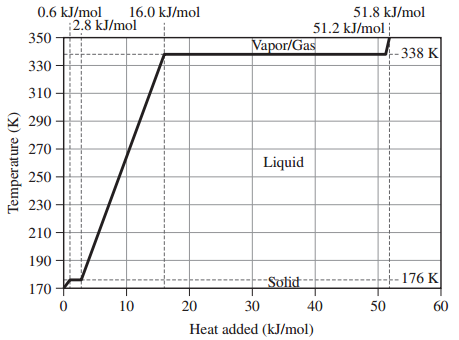# Problem: Assume that the values given here for 1 mole of methanol are constant over the relevant temperature ranges.Melting Point: 176 KBoiling Point: 338 KHfus: 2.2 kJ/mol KHvap: 35.2 kJ/mol KCs,solid: 105 J/mol KCs,liquid: 81.3 J/mol KCs,gas: 48 J/mol KGiven the heating curve for 1 mole of methanol beginning at 170 K and ending at 350 K answer the following questions:What is the value of qliquid for methanol?

###### FREE Expert Solution

Heat required to change the temperature of a substance without changing its physical state is given by:90% (470 ratings)###### Problem Details
Assume that the values given here for 1 mole of methanol are constant over the relevant temperature ranges.

Melting Point: 176 K

Boiling Point: 338 K

Hfus: 2.2 kJ/mol K

Hvap: 35.2 kJ/mol K

Cs,solid: 105 J/mol K

Cs,liquid: 81.3 J/mol K

Cs,gas: 48 J/mol K

Given the heating curve for 1 mole of methanol beginning at 170 K and ending at 350 K answer the following questions:What is the value of qliquid for methanol?